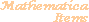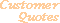Home Older Mathematica Items

Scientific Arts

Maxwellian Probability Distribution Function

Page 1 | Page 2 | Page 3 | Page 4

Inverse Series Method

An alternative to the point sampling numerical method is to perform series expansions of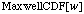and then invert these series using the InverseSeries function. These series need to be computed at several points to cover the region of interest. Here is an incomplete example: it almost works, but fails in certain cases. We leave it as a research project for the reader to improve upon this method so that it is more reliable.

Here is a series expansion of MaxwellCDF to third order about the point 1.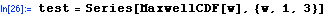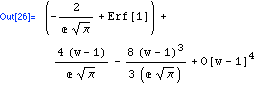The InverseSeries function can be used to invert this.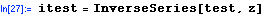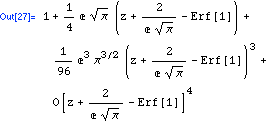The result of Series (or InverseSeries) is a SeriesData object, the first part of which is the expansion parameter, the second part the expansion point, and the third part is the list of coefficients (Mathematica formats this in the output to look like the standard representation of a series).

This is the expansion parameter for the inverse series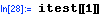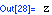This is the expansion point.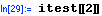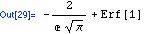And here is the list of coefficients in the series expansion of the inverse function.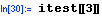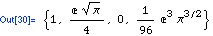Using this information we can write a function that generates the inverse series for the MaxwellCDF. With forethought we define it to return a list that has as its first element the expansion point and the series in the second element. Normal is used on the series to change it into a polynomial.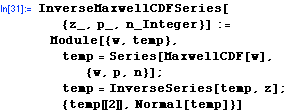Here is an example.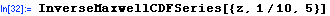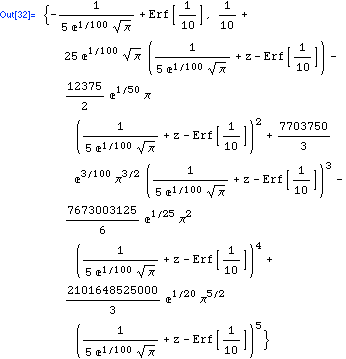This creates a table of these inverse series starting with a uniformly spaced set of points for the MaxwellCDFSeries. However, the resulting inverse series expansions' points are not uniformly spaced. This is no surprise: to get a uniformly spaced set of points we would need to have solved the inverse problem first.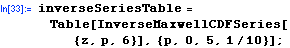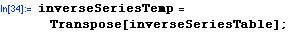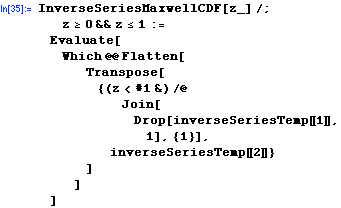Here is a timing comparison between the InverseSeries method and the InterpolatingFunction method. The InverseSeries approach is considerably slower.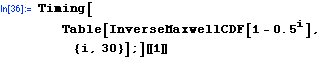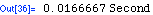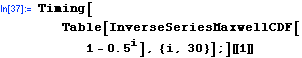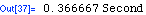Some of the speed loss can be recovered by compiling the function.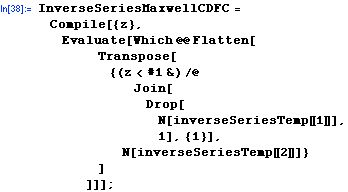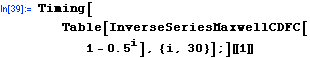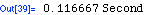Let's take a look at the functions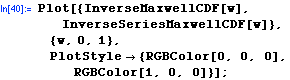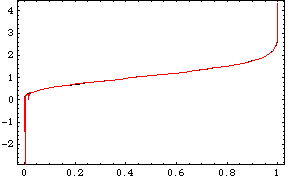There appear to be some numerical problems near zero.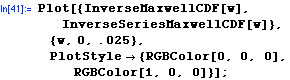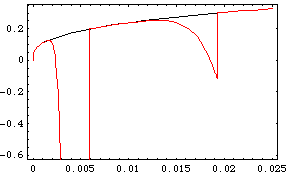The exercise for the interested reader is to track down the source of the numerical instability here. Presumably it is related to the radii of convergence of the inverse series that we have used.

<<PreviousNext >>

 For further information on our services send email to info@scientificarts.com . Contents of this web site Copyright © 1999-2011, Scientific Arts, LLC.
 s